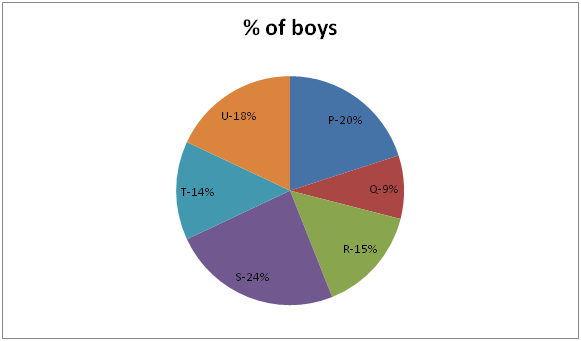# IBPS RRB Clerk/EPFO Assistant Prelims Quantitative Aptitude Questions 2019 (Day-10)

Dear Aspirants, Our IBPS Guide team is providing new series of Quantitative Aptitude Questions for IBPS RRB Clerk/EPFO Assistant Prelims 2019 so the aspirants can practice it on a daily basis. These questions are framed by our skilled experts after understanding your needs thoroughly. Aspirants can practice these new series questions daily to familiarize with the exact exam pattern and make your preparation effective.

##### EPFO Assistant Prelims Free Mock Test
[WpProQuiz 6721]

1) The ratio of upstream speed and downstream speed is 4: 5. If the speed of the stream is 9 kmph, find the distance covered in 2 hours upstream.

a) 144 km

b) 72 km

c) 288 km

d) 90 km

e) None of these

2) A train crosses a 450 m platform in 80 seconds and crosses a man in the platform in 20 seconds. Find the length of the train.

a) 200 m

b) 300 m

c) 450 m

d) 150 m

e) None of these

3) Pipe A and B can fill the tank in 36 h. If pipe A alone can fill the tank in 9 h, find the time taken by pipe B to empty the tank.

a) 13 h

b) 12 h

c) 18 h

d) 15 h

e) None of these

4) The ratio of investment of A and B is 1: 2 and the ratio of investment of B and C is 3: 4. If the difference between the profit of B and C is Rs600, find the total profit made by them after one year.

a) Rs5100

b) Rs2100

c) Rs3300

d) Rs4200

e) None of these

5) The ratio of ages of A and B 3 years ago is 2: 3 and after 3 years 3: 4. Find the average age of them.

a) 12

b) 15

c) 21

d) 18

e) None of these

Directions (6 – 10): Study the following information carefully and answer the questions given below.

The pie chart shows the percentage distribution of number of boys in six different schools.

Total number of boys = 24006) If the ratio between number of boys to number of girls in school “T” is 7: 8. Then find the number of girls in school “T”.

a) 324

b) 384

c) 336

d) 368

e) 372

7) Total number of boys in school “P” is what percent more than total number of boys in school “T”?

a) 33 (1/3) %

b) 66 (2/3) %

c) 38 (4/7) %

d) 42 (6/7) %

e) 50 %

8) Find the average number of boys in school Q, R and S together?

a) 384

b) 354

c) 324

d) 360

e) 408

9) Find the central angle of boys in school “U”?

a) 720

b) 68.20

c) 79.60

d) 57.20

e) 64.80

10) Total number of boys in school “P” is how much more then total number of boys in school “Q”?

a) 330

b) 264

c) 396

d) 363

e) 298

Direction (1-5) :

The ratio of upstream speed and downstream speed = 4: 5 (4x, 5x)

Speed of the stream = (5x – 4x)/2 = 0.5x

(i.e.) 9 = 0.5x

x = 18

Upstream speed = 18 * 4 = 72kmph

Required distance = 72 * 2 = 144 km

Let the speed and length of the train be x and y

Then

y + 450 = x * 5/18 * 80  —–(1)

y = x * 5/18 * 20   —- (2)

sub (2) in (1)

(x * 5/18 * 20) + 450 = x * 5/18 * 80

(x * 5/18 * 80) – (x * 5/18 * 20) = 450

x * 5/18 * 60 = 450

x = 27 kmph

y = 27 * 5/18 * 20 = 150 m

Pipe A and B ‘s one hour work,

A – B = 1/36

(i.e.) 1/9 – 1/x = 1/36

B ‘s one hour work,

1/x = 3/36 = 1/12

Pipe B alone empty the tank in 12 hours.

A and B = 1: 2

B and C = 3: 4

The ratio of A, B and C= 3: 6: 8

Total profit = 600/2 * 17 =Rs 5100

The ratio of ages of A and B 3 years ago = 2: 3 (2x, 3x)

(2x + 6)/(3x + 6) = ¾

8x + 24 = 9x + 18

x = 6

Age of A = 2 * 6 + 3 = 15years

Age of B = 3 * 6 + 3 = 21years

Average age = (15 + 21)/2 = 18years

Direction (6-10) :

Number of boys in school T = 2400 × 14/100 = 336

Ratio between number of boys to number of girls in school “T” = 7: 8

Number of girls in school T = 7x = 8 × 336

Number of girls in school T = 384

Required percentage = (20 – 14)/14 * 100

= 6/14 * 100

= 300/7 = 42 (6/7)% more

Average number of boys in school Q, R and S = (9 + 15 + 24)/3 = 16%

= 16/100 * 2400 = 384

Required angle = x * 100 = 18 * 360

Required angle = 64.80